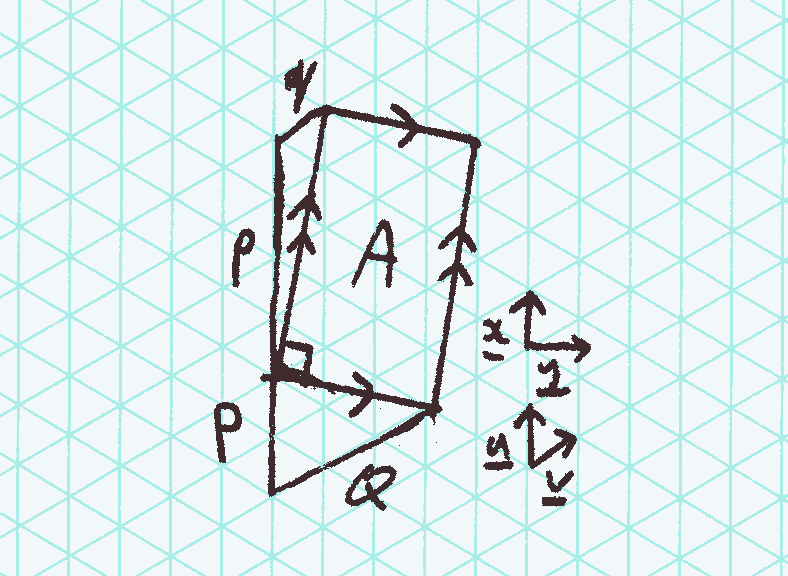# mathr / blog / #

## Rectangles on a triangular lattice

Starting from this post I'll be using MathJax to display prettier equations. It does require Javascript, but it's much less awkward than the alternatives I've considered.I was wondering about drawing rectangles on a regular triangular lattice. I found that the area is always a multiple of 4 cells.

Let $$\mathbf{u},\mathbf{v}$$ be the basis vectors of the triangular lattice, and $$\mathbf{x},\mathbf{y}$$ be the basis vectors of rectangular Cartesian coordinates. Specify a point on the lattice by $$p,q \in \mathbb{Z}$$, then:

$p \mathbf{u} + q \mathbf{v} = (p + \frac{q}{2}) \mathbf{x} + \frac{\sqrt{3}}{2} q \mathbf{y}$

with the squared Euclidean distance of the point from the origin being:

$\left|\left| p \mathbf{u} + q \mathbf{v} \right|\right| ^ 2= p^2 + q^2 + pq$

Now assume $$\gcd(p,q) = 1$$, to ensure that the line segment from the origin to the point passes through no other lattice points. A line $$(P,Q)$$ perpendicular to $$(p,q)$$ satisfies:

$(p + \frac{q}{2}) \mathbf{x} + \frac{\sqrt{3}}{2} q \mathbf{y} = \lambda ( -\frac{\sqrt{3}}{2} Q \mathbf{x} + (P + \frac{Q}{2}) \mathbf{y} )$

which reduces to

$\frac{P}{Q} = - \frac{p + 2 q}{2 p + q}$

Letting $$K = \gcd(p + 2 q, 2 p + q)$$, then $$P = -\frac{p + 2 q}{K}$$ and $$Q = \frac{2 p + q}{K}$$ and $$\gcd(P,Q) = 1$$.

The area of the rectangle can be found in terms of $$p$$ and $$q$$:

$A^2 = (p^2 + q^2 + pq) (P^2 + Q^2 + PQ)$

which reduces to

$A = \sqrt{3} \frac{p^2 + q^2 + pq}{K}$

Expressing this in terms of the smallest triangular cell on the lattice, which has area $$\frac{\sqrt{3}}{4}$$, gives a count of triangles:

$N = 4 \frac{p^2 + q^2 + pq}{K}$

Recall that $$\gcd(p,q) = 1$$ and $$\gcd(p + 2 q, 2 p + q) = K$$. This means that $$K \mid p + 2 q$$ and $$K \mid 2 p + q$$ which implies that $$K \mid 3 (p + q)$$. So $$K \mid 3$$ or $$K \mid p + q$$. When $$K$$ doesn't divide $$p + q$$ then $$K \in \{1,3\}$$. Suppose $$K \mid p + q$$, then $$K \mid p + 2 q$$ implies that $$K \mid q$$, and similarly $$K \mid 2 p + q$$ implies that $$K \mid p$$. But $$\gcd(p,q) = 1$$, therefore $$K = 1$$.

$$K = 1$$ implies that $$4 \mid N$$, so it remains to consider the other case when $$K = 3$$. Let $$p = 3 a + b$$ and $$q = 3 c + d$$ with $$b,d \in \{0,1,2\}$$. Now $$K \mid b + 2 d$$ and $$K \mid 2 b + d$$. Exhaustive case analysis shows that this holds only when $$b = d$$. Expanding

$p^2 + q^2 + pq = 3(\ldots) + b^2 + d^2 + bd = 3(\ldots) + 3 b^2$

shows that $$3 \mid p^2 + q^2 + pq$$. So $$\frac{p^2 + q^2 + pq}{K}\ \in \mathbb{Z}$$ and $$4 \mid N$$: the area of a rectangle on a triangular lattice is always a multiple of 4 cells.